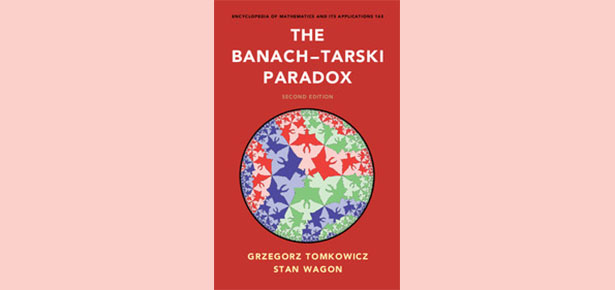Products and services

# Fifteen Eighty Four

5
Apr
2017

## It Is Best to Accept the Banach Tarski Paradox

Stan WagonIn October 2016, a few months after our book appeared, I was at an AMS conference in Denver where Andreas Blass told me of an interesting fact related to the Banach–Tarski Paradox (BTP). I will summarize the story here.

Many people, upon hearing about the BTP (a ball may be partitioned into five pieces that can be moved in space to form two balls, each the same size of the first), think that such an assertion is absurd and must surely be discarded from any reasonable foundation of mathematics. For one example, here is what noted physicist and mathematician Roger Penrose has said about this: “It’s not altogether uncontroversial that the axiom of choice should be accepted as something that is universally valid. My own position is to be cautious about it. The trouble with this axiom is that it is a pure ‘existence’ assertion, without any hint of a rule whereby the set might be specified. In fact, it has a number of alarming consequences. One of these is the Banach–Tarski theorem.” [R. Penrose, The Road to Reality, Knopf, 2005, pp. 14–15]

The pieces in the BTP are nonmeasurable and mathematicians have learned to live with nonmeasurable sets. But it is in fact possible to eliminate nonmeasurable sets by discarding the Axiom of Choice (AC) and assuming that all subsets of R^n are Lebesgue measurable; call this assertion LM. Then LM violates AC, but the theory ZF + LM  is known to be consistent (ZF here is Zermelo–Fraenkel set theory, without the Axiom of Choice), so let us imagine for a moment that we are taking ZF + LM as the foundation of mathematics.

It turns out that ZF + LM implies that a certain paradox exists that is just as troubling as the BTP, if not more so. To set the stage, consider the National Football League, which has 32 teams and 53 · 32 = 1696 players. If the players were assigned to teams in some other way, subject only to the conditions that a team cannot have zero players and each player can be on only one team, then there can easily be more than 32 teams. But could there be more than 1696 teams? Of course not. The idea of grouping players into nonempty teams so that there are more teams than players is ludicrous. Yet this is the essence of the phenomenon that Blass told me about: this sort of thing can arise in a world without the Axiom of Choice.

In the theory ZF + LM, this happens: consider the players to be the real numbers and the teams to be the sets of real numbers that differ by a rational number. So one team might be {π, π + 1, π + 1/2 , π – 17/4 ,…}. That is, we are working with R and R / Q. Now, we have two remarkable results (recall that cardinality is defined in the usual way: |X| ≤ |Y| , means that there is a one-one function from X to Y ; |X| = |Y| means that |X| ≤ |Y| and |Y| ≤ |X|, and then the classic Schröder–Bernstein Theorem, which is quite constructive, implies that there is a bijection from X to Y ).

Theorem 1 (Mycielski, 1964).  In ZF, |R| ≤ |R / Q|.
Theorem 2 (Sierpiński, 1916).  In ZF + LM, |R / Q| ≰  |R|

Taken together, these mean that in ZF + LM, we have |R| < |R / Q|. This means that the two sets— the “teams” R / Q and the “players” R—are comparable as regards cardinality, but the comparison goes in a way that defies intuition: there are more teams than players!

Another way of looking at it is this. In ZF, cardinality is a partial ordering, so for R and R / ℚ there are four possibilities:
1.  |R| = |R / Q|
2. R and R / Q are incomparable: |R / Q| ≰ |R| and |R| ≰ |R / Q|
3.  |R / Q| < |R|
4.  |R| < |R / Q|

Possibility (1) is certainly viable, since it follows from AC. But Theorem 1 says that (2) and (3) cannot happen. So the two sets are comparable and if (1) fails, (4) must hold. But (4) says that the ostensibly smaller set, R / Q which is made by grouping reals together into “rational teams,” is actually larger. And Theorems 1 and 2 together show that (4) can happen.

I am just finishing a paper with Alan Taylor that will give details of the proofs of these two theorems (they are quite short), discuss how they relate to other areas of mathematics, and contain our opinions on what these results mean for the foundations of set theory. Not surprisingly, we feel that these results support the view that one should use ZF + AC as the foundational basis for mathematics. One can formulate an assertion, the Weak Partition Principle, which says that if Y is a family of disjoint nonempty subsets of X, then |X| ≮ |Y|; in short, there cannot be more teams than players. The results above show that ZF is not strong enough to prove WPP and that ZF + LM actually negates WPP. But we believe WPP to be obvious (of course, it follows from the Axiom of Choice), and so these results show that ZF is inadequate as a foundation for mathematics.

If you wish to see a preprint of our paper, just contact me at [email protected].